# Notes on making scatterplots in matplotlib and seaborn

Many of my programming tips, like my notes for making Leaflet maps in R or margins plots in Stata, I’ve just accumulated doing projects over the years. My current workplace is a python shop though, so I am figuring it out all over for some of these things in python. I made some ugly scatterplots for a presentation the other day, and figured it would be time to spend alittle time making some notes on making them a bit nicer.

For prior python graphing post examples, I have:

For this post, I am going to use the same data I illustrated with SPSS previously, a set of crime rates in Appalachian counties. Here you can download the dataset and the python script to follow along.

# Making scatterplots using matplotlib

So first for the upfront junk, I load my libraries, change my directory, update my plot theme, and then load my data into a dataframe crime_dat. I technically do not use numpy in this script, but soon as I take it out I’m guaranteed to need to use np. for something!

################################################################
import pandas as pd
import numpy as np
import os
import matplotlib
import matplotlib.pyplot as plt
import seaborn as sns

my_dir = r'C:\Users\andre\OneDrive\Desktop\big_scatter'
os.chdir(my_dir)

andy_theme = {'axes.grid': True,
'grid.linestyle': '--',
'legend.framealpha': 1,
'legend.facecolor': 'white',
'legend.fontsize': 14,
'legend.title_fontsize': 16,
'xtick.labelsize': 14,
'ytick.labelsize': 14,
'axes.labelsize': 16,
'axes.titlesize': 20,
'figure.dpi': 100}

matplotlib.rcParams.update(andy_theme)
################################################################

First, lets start from the base scatterplot. After defining my figure and axis objects, I add on the ax.scatter by pointing the x and y’s to my pandas dataframe columns, here Burglary and Robbery rates per 100k. You could also instead of starting from the matplotlib objects start from the pandas dataframe methods (as I did in my prior histogram post). I don’t have a good reason for using one or the other.

Then I set the axis grid lines to be below my points (is there a way to set this as a default?), and then I set my X and Y axis labels to be nicer than the default names.

################################################################
#Default scatterplot
fig, ax = plt.subplots(figsize=(6,4))
ax.scatter(crime_dat['burg_rate'], crime_dat['rob_rate'])
ax.set_axisbelow(True)
ax.set_xlabel('Burglary Rate per 100,000')
ax.set_ylabel('Robbery Rate per 100,000')
plt.savefig('Scatter01.png', dpi=500, bbox_inches='tight')
plt.show()
################################################################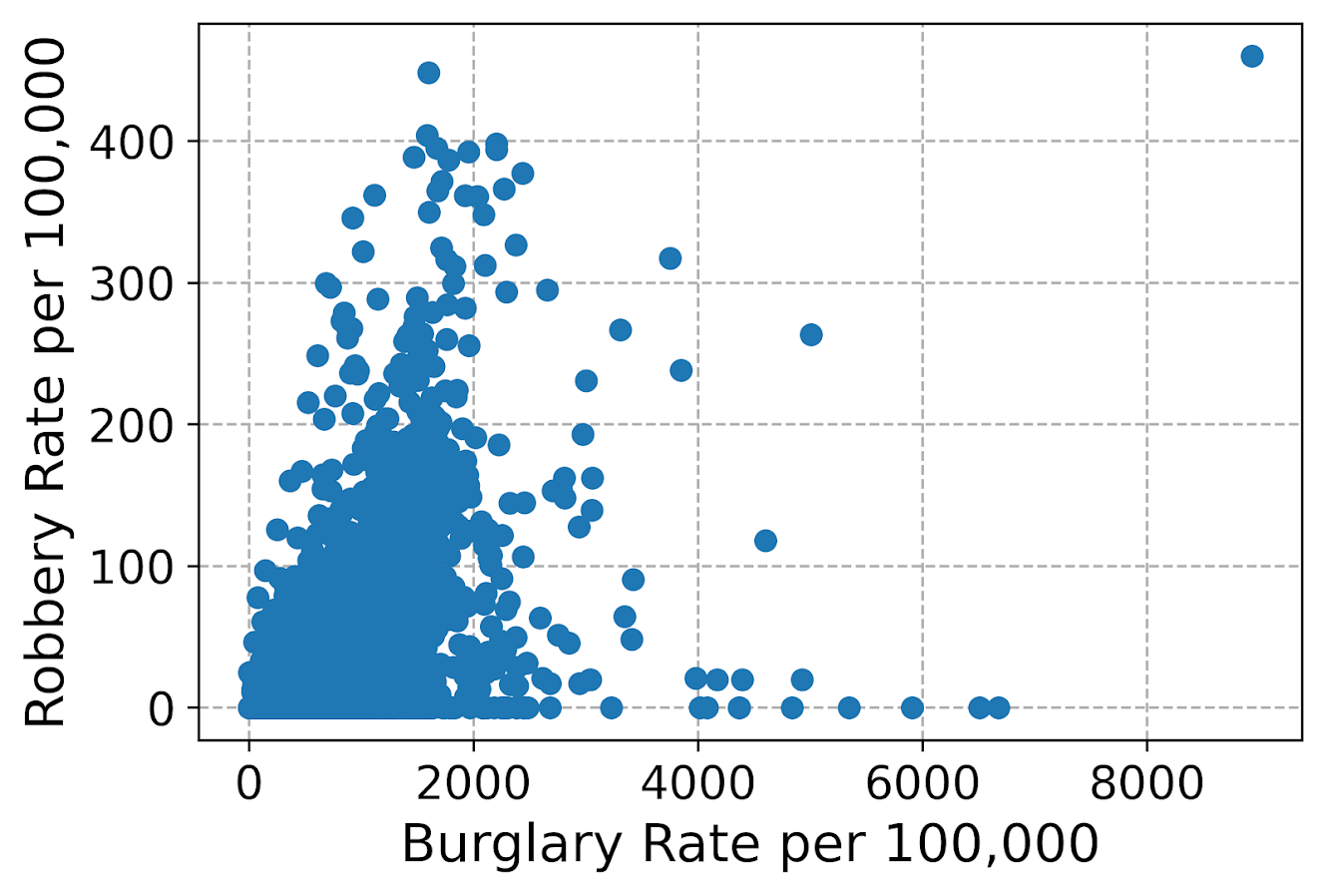You can see here the default point markers, just solid blue filled circles with no outline, when you get a very dense scatterplot just looks like a solid blob. I think a better default for scatterplots is to plot points with an outline. Here I also make the interior fill slightly transparent. All of this action is going on in the ax.scatter call, all of the other lines are the same.

################################################################
#Making points have an outline and interior fill
fig, ax = plt.subplots(figsize=(6,4))
ax.scatter(crime_dat['burg_rate'], crime_dat['rob_rate'],
c='grey', edgecolor='k', alpha=0.5)
ax.set_axisbelow(True)
ax.set_xlabel('Burglary Rate per 100,000')
ax.set_ylabel('Robbery Rate per 100,000')
plt.savefig('Scatter02.png', dpi=500, bbox_inches='tight')
plt.show()
################################################################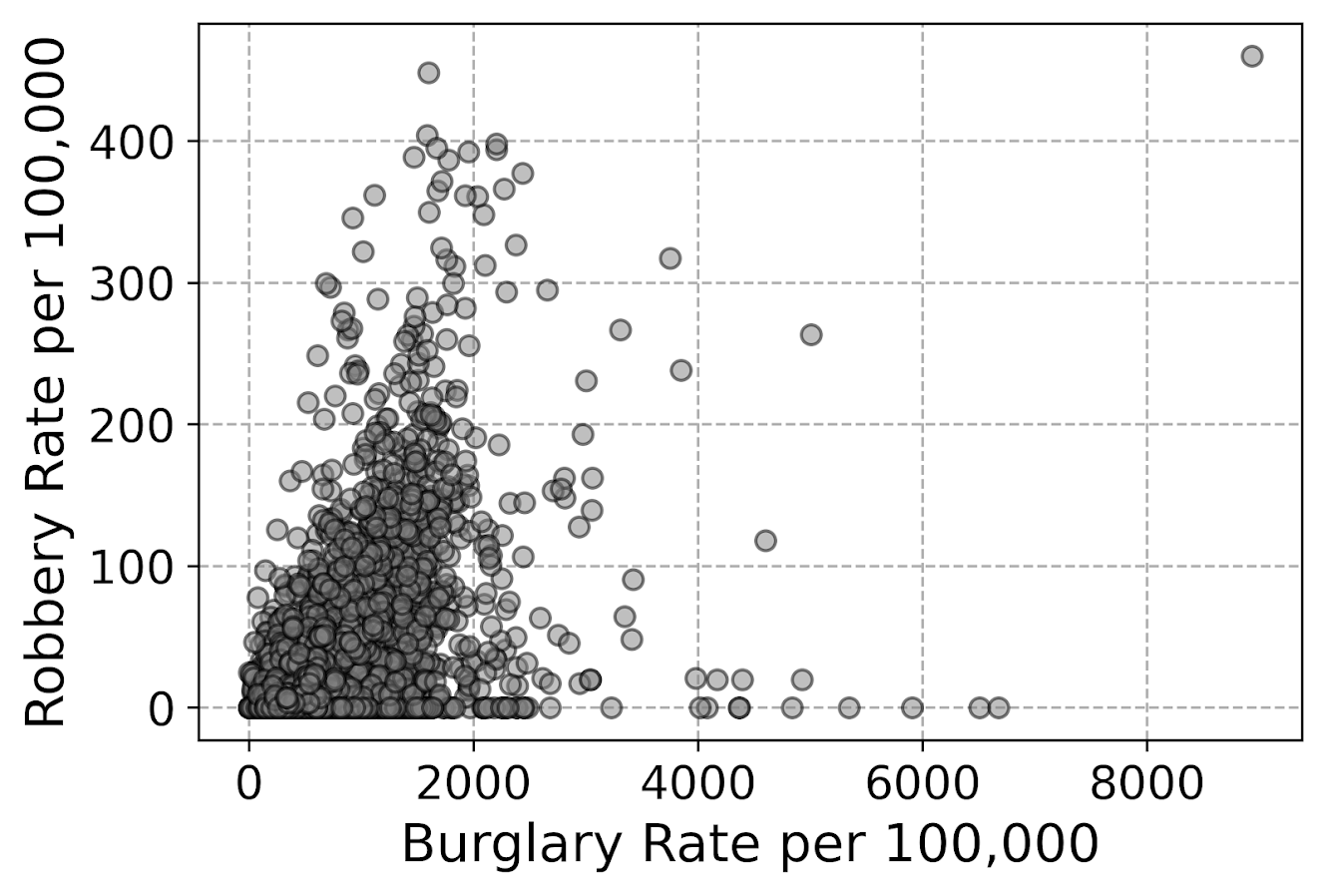So that is better, but we still have quite a bit of overplotting going on. Another quick trick is to make the points smaller and up the transparency by setting alpha to a lower value. This allows you to further visualize the density, but then makes it a bit harder to see individual points – if you started from here you might miss that outlier in the upper right.

Note I don’t set the edgecolor here, but if you want to make the edges semitransparent as well you could do edgecolor=(0.0, 0.0, 0.0, 0.5), where the last number of is the alpha transparency tuner.

################################################################
#Making the points small and semi-transparent
fig, ax = plt.subplots(figsize=(6,4))
ax.scatter(crime_dat['burg_rate'], crime_dat['rob_rate'], c='k',
alpha=0.1, s=4)
ax.set_axisbelow(True)
ax.set_xlabel('Burglary Rate per 100,000')
ax.set_ylabel('Robbery Rate per 100,000')
plt.savefig('Scatter03.png', dpi=500, bbox_inches='tight')
plt.show()
################################################################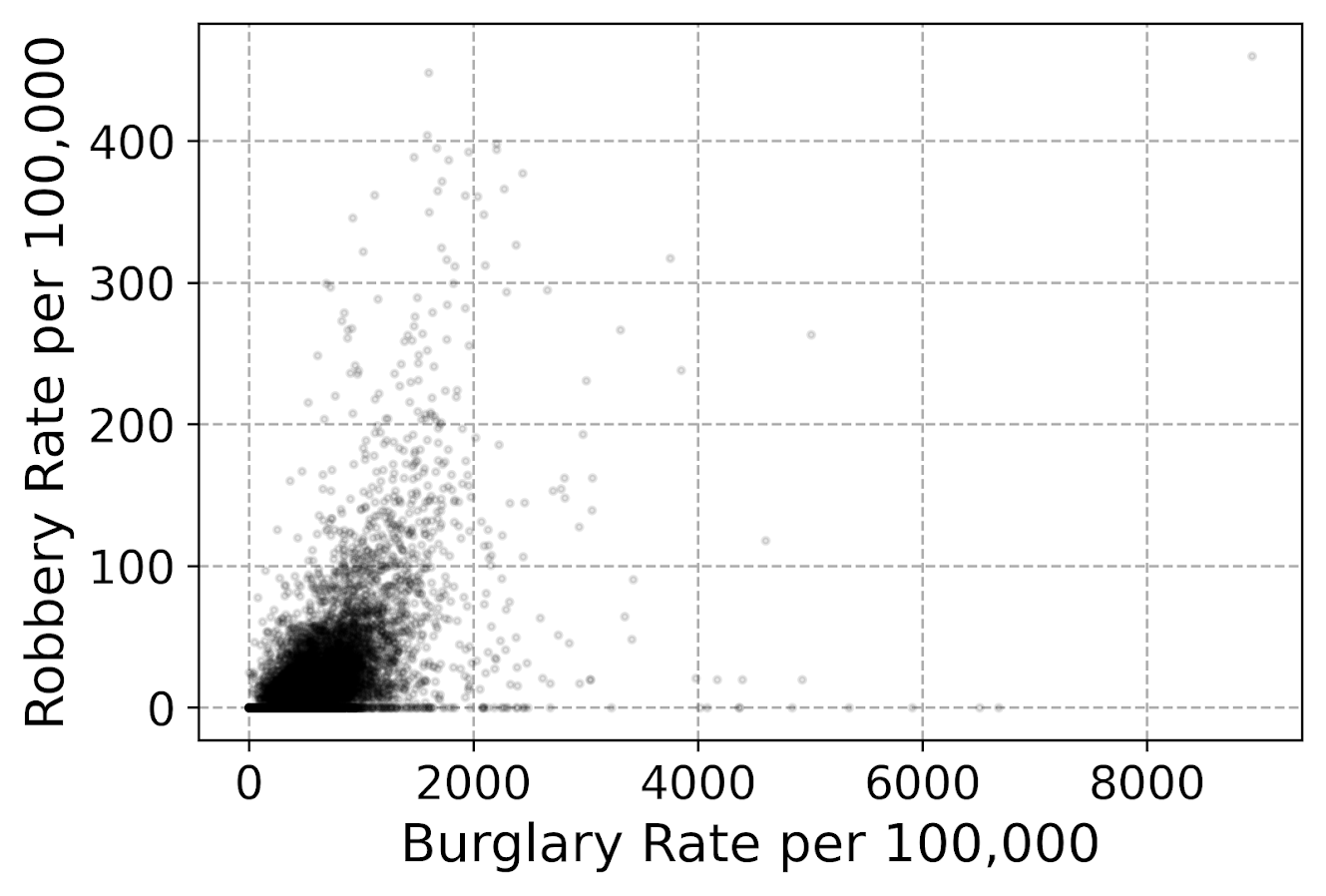This dataset has around 7.5k rows in it. For most datasets of anymore than a hundred points, you often have severe overplotting like you do here. One way to solve that problem is to bin observations, and then make a graph showing the counts within the bins. Matplotlib has a very nice hexbin method for doing this, which is easier to show than explain.

################################################################
#Making a hexbin plot
fig, ax = plt.subplots(figsize=(6,4))
hb = ax.hexbin(crime_dat['burg_rate'], crime_dat['rob_rate'],
gridsize=20, edgecolors='grey',
cmap='inferno', mincnt=1)
ax.set_axisbelow(True)
ax.set_xlabel('Burglary Rate per 100,000')
ax.set_ylabel('Robbery Rate per 100,000')
cb = fig.colorbar(hb, ax=ax)
plt.savefig('Scatter04.png', dpi=500, bbox_inches='tight')
plt.show()
################################################################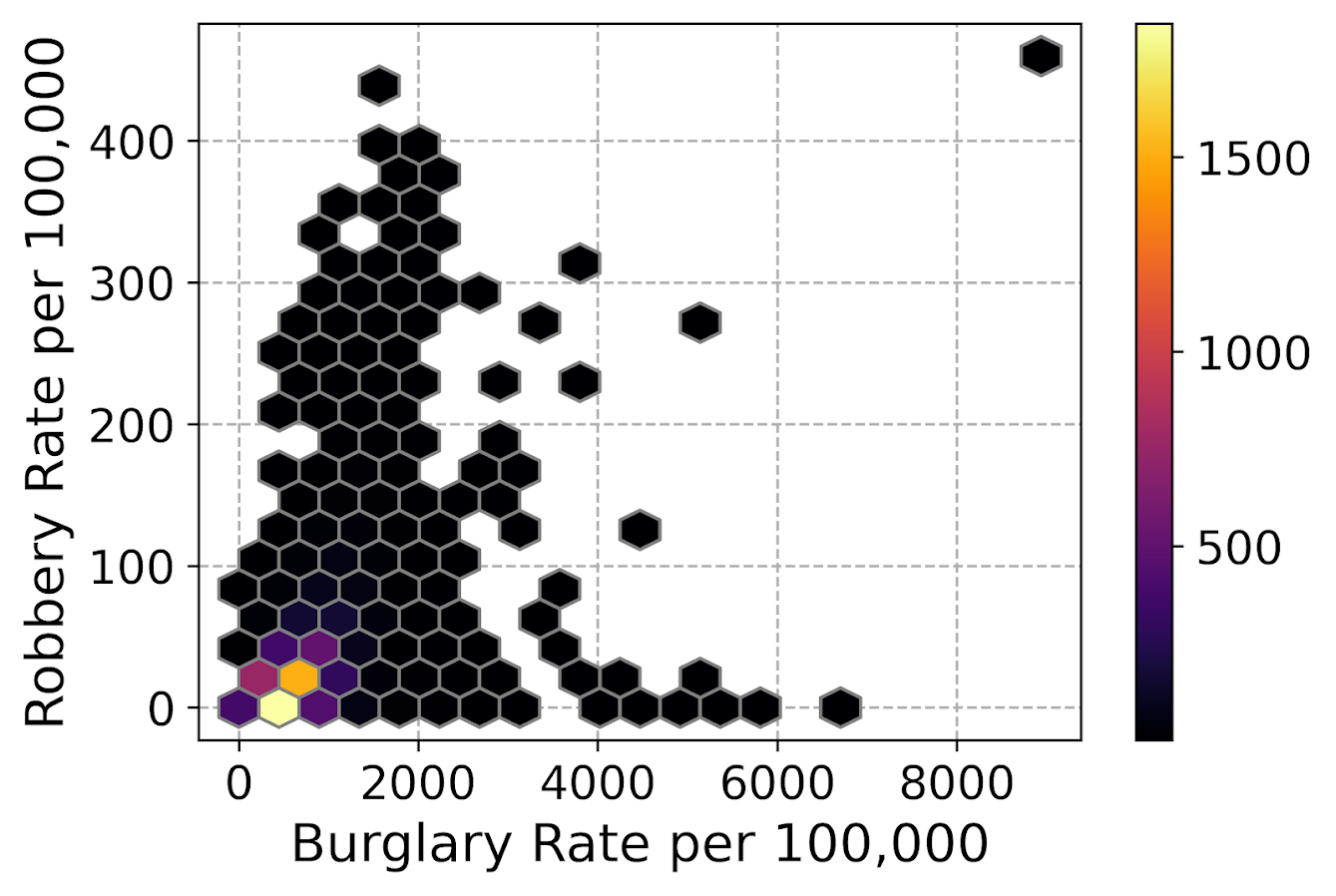So for the hexbins I like using the mincnt=1 option, as it clearly shows areas with no points, but then you can still spot the outliers fairly easy. (Using white for the edge colors looks nice as well.)

You may be asking, what is up with that outlier in the top right? It ends up being Letcher county in Kentucky in 1983, which had a UCR population estimate of only 1522, but had a total of 136 burglaries and 7 robberies. This could technically be correct (only some local one cop town reported, and that town does not cover the whole county), but I’m wondering if this is a UCR reporting snafu.

It is also a good use case for funnel charts. I debated on making some notes here about putting in text labels, but will hold off for now. You can add in text by using ax.annotate fairly easy by hand, but it is hard to automate text label positions. It is maybe easier to make interactive graphs and have a tooltip, but that will need to be another blog post as well.

# Making scatterplots using seaborn

The further examples I show are using the seaborn library, imported earlier as sns. I like using seaborn to make small multiple plots, but it also has a very nice 2d kernel density contour plot method I am showing off.

Note this does something fundamentally different than the prior hexbin chart, it creates a density estimate. Here it looks pretty but creates a density estimate in areas that are not possible, negative crime rates. (There are ways to prevent this, such as estimating the KDE on a transformed scale and retransforming back, or reflecting the density back inside the plot would probably make more sense here, ala edge weighting in spatial statistics.)

Here the only other things to note are used filled contours instead of just the lines, I also drop the lowest shaded area (I wish I could just drop areas of zero density, note dropping the lowest area drops my outlier in the top right). Also I have a tough go of using the default bandwidth estimators, so I input my own.

################################################################
#Making a contour plot using seaborn
g = sns.kdeplot(crime_dat['burg_rate'], crime_dat['rob_rate'],
g.set_axisbelow(True)
g.set_xlabel('Burglary Rate per 100,000')
g.set_ylabel('Robbery Rate per 100,000')
plt.savefig('Scatter05.png', dpi=500, bbox_inches='tight')
plt.show()
################################################################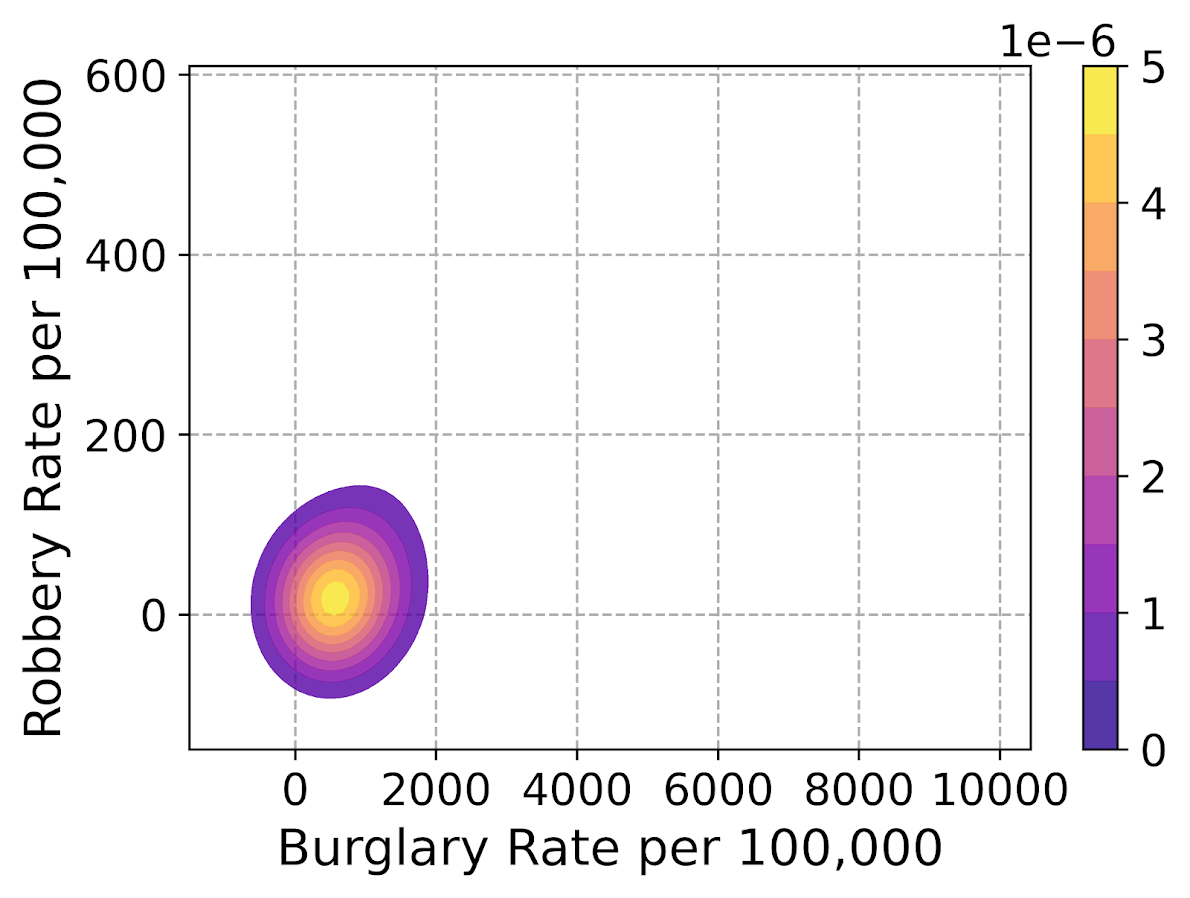So far I have not talked about the actual marker types. It is very difficult to visualize different markers in a scatterplot unless they are clearly separated. So although it works out OK for the Iris dataset because it is small N and the species are clearly separated, in real life datasets it tends to be much messier.

So I very rarely use multiple point types to symbolize different groups in a scatterplot, but prefer to use small multiple graphs. Here is an example of turning my original scatterplot, but differentiating between different county areas in the dataset. It is a pretty straightforward update using sns.FacetGrid to define the group, and then using g.map. (There is probably a smarter way to set the grid lines below the points for each subplot than the loop.)

################################################################
#Making a small multiple scatterplot using seaborn
g = sns.FacetGrid(data=crime_dat, col='subrgn',
col_wrap=2, despine=False, height=4)
g.map(plt.scatter, 'burg_rate', 'rob_rate', color='grey',
s=12, edgecolor='k', alpha=0.5)
g.set_titles("{col_name}")
for a in g.axes:
a.set_axisbelow(True)
g.set_xlabels('Burglary Rate per 100,000')
g.set_ylabels('Robbery Rate per 100,000')
plt.savefig('Scatter06.png', dpi=500, bbox_inches='tight')
plt.show()
################################################################And then finally I show an example of making a small multiple hexbin plot. It is alittle tricky, but this is an example in the seaborn docs of writing your own sub-plot function and passing that.

To make this work, you need to pass an extent for each subplot (so the hexagons are not expanded/shrunk in any particular subplot). You also need to pass a vmin/vmax argument, so the color scales are consistent for each subplot. Then finally to add in the color bar I just fiddled with adding an axes. (Again there is probably a smarter way to scoop up the plot coordinates for the last plot, but here I just experimented till it looked about right.)

################################################################
#Making a small multiple hexbin plot using seaborn

#https://stackoverflow.com/a/31385996/604456
def loc_hexbin(x, y, **kwargs):
kwargs.pop("color", None)
plt.hexbin(x, y, gridsize=20, edgecolor='grey',
cmap='inferno', mincnt=1,
vmin=1, vmax=700, **kwargs)

g = sns.FacetGrid(data=crime_dat, col='subrgn',
col_wrap=2, despine=False, height=4)
g.map(loc_hexbin, 'burg_rate', 'rob_rate',
edgecolors='grey', extent=[0, 9000, 0, 500])
g.set_titles("{col_name}")
for a in g.axes:
a.set_axisbelow(True)
#This goes x,y,width,height
cax = g.fig.add_axes([0.55, 0.09, 0.03, .384])
plt.colorbar(cax=cax, ax=g.axes)
g.set_xlabels('Burglary Rate per 100,000')
g.set_ylabels('Robbery Rate per 100,000')
plt.savefig('Scatter07.png', dpi=500, bbox_inches='tight')
plt.show()
################################################################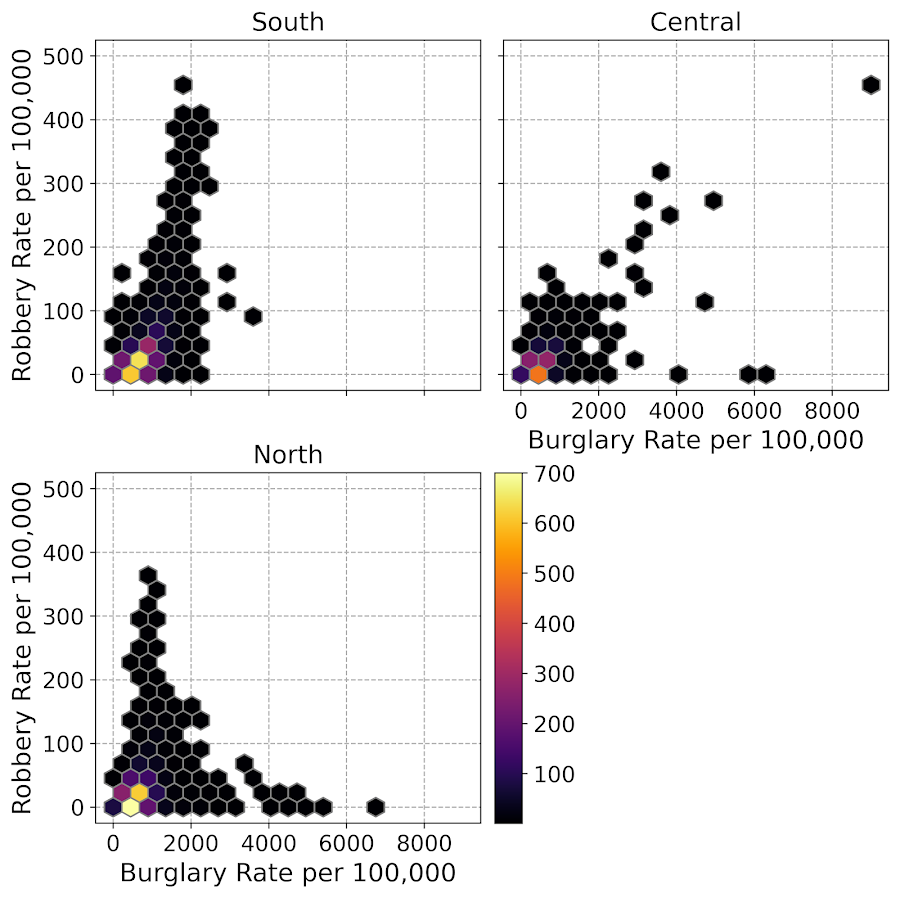Another common task with scatterplots is to visualize a smoother, e.g. E[Y|X] the expected mean of Y conditional on X, or you could do any other quantile, etc. That will have to be another post though, but for examples I have written about previously I have jittering 0/1 data, and visually weighted regression.